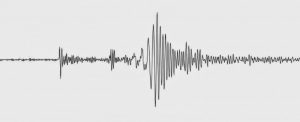# Prediction of large earthquake probability improvedAs part of the “Research in Collaborative Mathematics” project run by the Obra Social “la Caixa,” researchers of the Mathematics Research Centre (CRM) and the UAB have developed a mathematical law to explain the size distribution of earthquakes, even in the cases of large-scale earthquakes such as those which occurred in Sumatra (2004) and in Japan (2011).

The probability of an earthquake occurring exponentially decreases as its magnitude value increases. Fortunately, mild earthquakes are more probable than devastatingly large ones. This relation between probability and earthquake magnitude follows a mathematical curve called the Gutenberg-Richter law, and helps seismologists predict the probabilities of an earthquake of a specific magnitude occurring in some part of the planet.

The law however lacks the necessary tools to describe extreme situations. For example, although the probability of an earthquake being of the magnitude of 12 is zero, since technically this would imply the earth breaking in half, the mathematics of the Gutenberg-Richter law do not consider impossible a 14-magnitude earthquake.

“The limitations of the law are determined by the fact that the Earth is finite, and the law describes ideal systems, in a planet with an infinite surface,” explains Isabel Serra, first author of the article, researcher at CRM and affiliate lecturer of the UAB Department of Mathematics.

To overcome these shortages, researchers studied a small modification in the Gutenberg-Richter law, a term which modified the curve precisely in the area in which probabilities were the smallest. “This modification has important practical effects when estimating the risks or evaluating possible economic losses. Preparing for a catastrophe where the losses could be, in the worst of the cases, very high in value, is not the same as not being able to calculate an estimated maximum value,” clarifies co-author Álvaro Corral, researcher at the Mathematics Research Centre and the UAB Department of Mathematics.

Recommended For You  First detailed simulation of 2004 megathrust earthquake

Obtaining the mathematical curve which best fits the registered data on earthquakes is not an easy task when dealing with large tremors. From 1950 to 2003 there were only seven earthquakes measuring higher than 8.5 on the Richter scale and since 2004 there have only been six. Although we are now in a more active period following the Sumatra earthquake, there are very few cases and that makes it statistically a poorer period. Thus, the mathematical treatment of the problem becomes much more complex than when there is an abundance of data. For Corral, “this is where the role of mathematics is fundamental to complement the research of seismologists and guarantee the accuracy of the studies.” According to the researcher, the approach currently used to analyse seismic risk is not fully correct and, in fact, there are many risk maps which are downright incorrect, “which is what happened with the Tohoku earthquake of 2011, where the area contained an under-dimensioned risk.” “Our approach has corrected some things, but we are still far from being able to give correct results in specific regions,” Corral continues.

The mathematical expression of the law at the seismic moment, proposed by Serra and Corral, meets all the conditions needed to determine both the probability of smaller earthquakes and of large ones, by adjusting itself to the most recent and extreme cases of Tohoku, in Japan (2011) and Sumatra, in Indonesia (2004); as well as to determine negligible probabilities for earthquakes of disproportionate magnitudes.

The derived Gutenberg-Richter law has also been used to begin to explore its applications in the financial world. Isabel Serra worked in this field before beginning to study earthquakes mathematically. “The risk assessment of a firm’s economic losses is a subject insurance companies take very seriously, and the behaviour is similar: the probability of suffering losses decreases in accordance with the increase in volume of losses, according to a law that is similar to that of Gutenberg-Richter, but there are limit values which these laws do not take into consideration, since no matter how big the amount, the probability of losses of that amount never results in zero” Serra explains. “That makes the ‘expected value of losses’ enormous. To solve this, changes would have to be made to the law similar to those we introduced to the law on earthquakes.”

Recommended For You  Mine landslide triggered quakes

Reference:
Isabel Serra, Álvaro Corral. Deviation from power law of the global seismic moment distribution. Scientific Reports, 2017; 7: 40045 DOI: 10.1038/srep40045

Note: The above post is reprinted from materials provided by Universitat Autònoma de Barcelona.

SHARE